### Vol 17, No 12 (2017) / Christodoulou

A physical interpretation of the Titius-Bode rule and its connection to the closed orbits of Bertrandʼs theorem

# A physical interpretation of the Titius-Bode rule and its connection to the closed orbits of Bertrandʼs theorem

Christodoulou Dimitris M.1, 2, , Kazanas Demosthenes3,

Lowell Center for Space Science and Technology, University of Massachusetts Lowell, Lowell, MA 01854, USA
Department of Mathematical Sciences, University of Massachusetts Lowell, Lowell, MA 01854, USA
NASA Goddard Space Flight Center, Laboratory for High-Energy Astrophysics, Code 663, Greenbelt, MD 20771, USA

† Corresponding author. E-mail: dimitris_christodoulou@uml.edu demos.kazanas@nasa.gov

Abstract: Abstract

We consider the geometric Titius-Bode rule for the semimajor axes of planetary orbits. We derive an equivalent rule for the midpoints of the segments between consecutive orbits along the radial direction and we interpret it physically in terms of the work done in the gravitational field of the Sun by particles whose orbits are perturbed around each planetary orbit. On such energetic grounds, it is not surprising that some exoplanets in multiple-planet extrasolar systems obey the same relation. However, it is surprising that this simple interpretation of the Titius-Bode rule also reveals new properties of the bound closed orbits predicted by Bertrand’s theorem, which has been known since 1873.

Keywords: planets and satellites: formation;planets and satellites: general;protoplanetary disks

## 1 Introduction

The numerical algorithm called the Titius-Bode “law” has been known for 250 years (e.g., Nieto ; Lecar ; Danby ; Murray & Dermott ). It relies on an ad-hoc geometric progression to describe the positions of the planets in the solar system and works fairly well out to Uranus but no farther (Jaki ). The same phenomenology has also been applied to the satellites of the gaseous giant planets (Neuhaeuser & Feitzinger ; Murray & Dermott ). Two modern brief reviews of the history along with criticisms of this rule have been written by Graner & Dubrulle () and Hayes & Tremaine (). Currently, the general consensus is that a satisfactory physical basis has not been found for this numerical coincidence despite serious efforts by many researchers over the past three centuries. Furthermore, opinions differ on whether such a physical basis exists at all.

Apparently, many researchers still believe that the Titius-Bode algorithm does have a physical foundation and continue to work on this problem. In particular, the last decade of the twentieth century saw a resurgence of investigations targeting precisely two questions: the origin of the “law” (Graner & Dubrulle ; Dubrulle & Graner ; Li et al. ; Nottale et al. ; Laskar ) and its statistical robustness against the null hypothesis (Hayes & Tremaine ; Murray & Dermott ; Lynch ). Furthermore, in this century, some extrasolar systems have been discovered in which the planets appear to obey the Titius-Bode rule and the rule is used as a predictor of additional planets yet to be discovered in these multiple-planet systems (Poveda & Lara , Poveda & Lara , Bovaird & Lineweaver ; Huang & Bakos ; Bovaird et al. ).

In Section 2, we examine the Titius-Bode rule in its original form, which is a geometric progression of the semimajor axes of most planetary orbits in the solar system. By inductive reasoning, we associate the geometric rule with the work done in the gravitational field of the Sun by perturbed particles orbiting in the vicinity of planetary orbits, but we find that the spacing of the semimajor axes is not the right qualifier for the physical profile dictated by the Sun’s gravitational potential. Then we derive another rule for a group of hypothetical orbits that are equally spaced between the actual semimajor axes and we interpret this rule physically in terms of the gravitational potential differences of particles perturbed around the actual orbits of the planets. Our results support the discovery of Laskar () (for related recent works see Jiang et al. ; Laskar & Petit ) that such an arrangement of orbits implies that protoplanets do not interfere with one another during their formation stage, thus a planet is expected to be formed at every available orbit in the geometric progression. Furthermore, our results reveal new geometric properties (see the Appendix) of the bound closed orbits predicted in spherical potentials by the celebrated theorem of Bertrand (). In Section 3, we summarize and discuss these results.

## 2 Titius-Bode Rule Rewritten and Interpreted Physically

In its original form, the Titius-Bode rule dictates that the semimajor axes of most planetary orbits follow a geometric progression. (In some forms, an additional term of 0.4 is added ad hoc in order to reproduce the innermost three planets that appear to be in arithmetic progression.) The geometric progression is described formally by two equivalent relations: Consider three consecutive orbits with semimajor axes a 1, a 2 and a 3 (Fig. 1); then the intermediate axis must be the geometric mean of its neighboring axes, viz.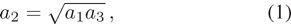or equivalently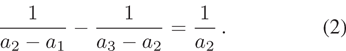The form of Equation (2) contains reciprocal distances and this is a sufficient hint that the relation could be associated with the central gravitational potential due to the Sun. But, as illustrated in Figure 1, such a simple association is not entirely straightforward because the distances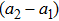and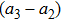are not central, i.e., they are not measured from the Sun.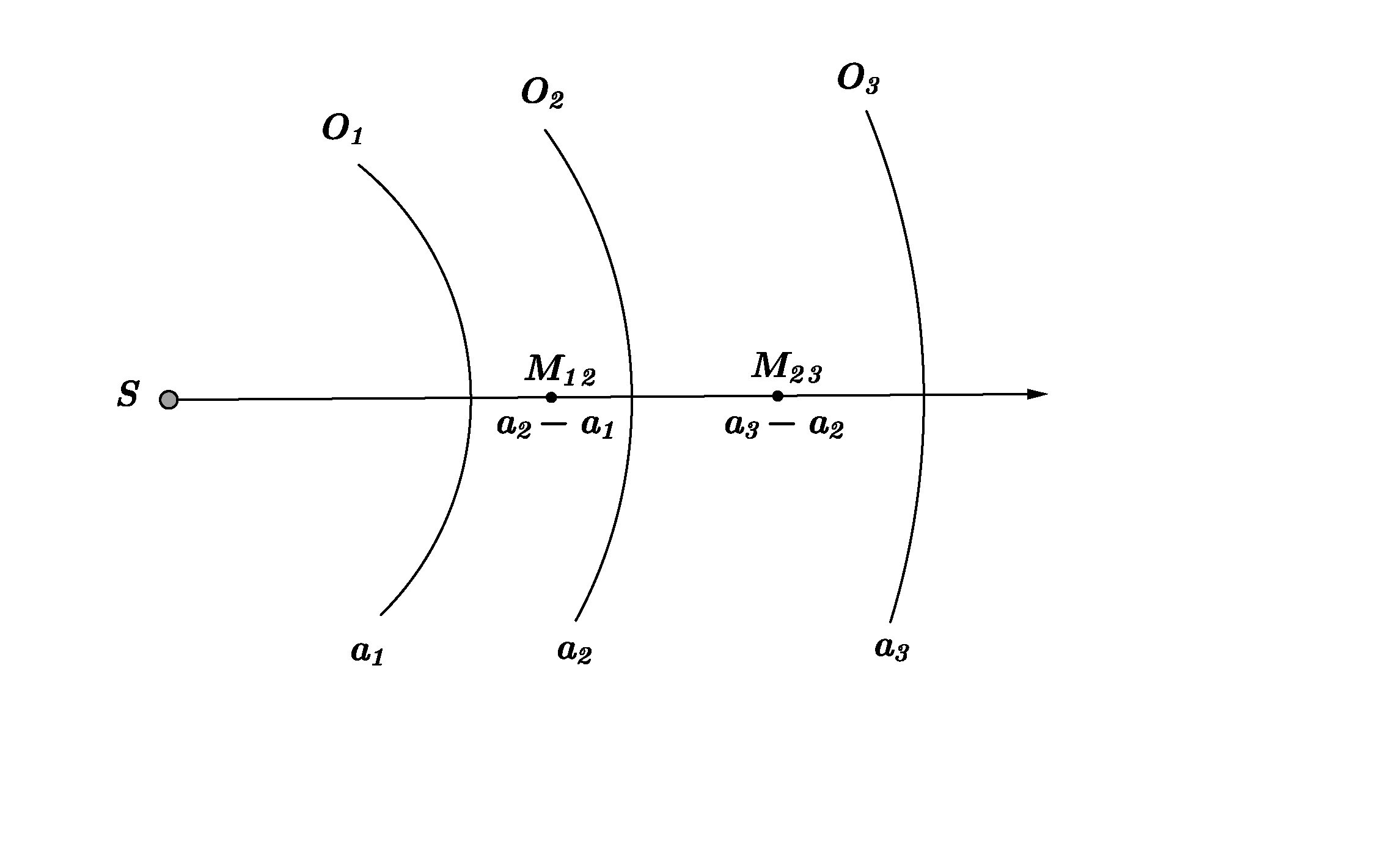Fig. 1. Schematic diagram of three consecutive planetary orbits with semimajor axes a 1, a 2 and a 3 in geometric progression (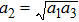). The midpoints M 12 and M 23 of the segments between the orbits are also marked along the ray from the Sun S.

In order to recast the rule in terms of central reciprocal distances, we define hypothetical orbits that are equidistant between the semimajor axes. In Figure 1, such orbits would cross the ray from S at the midpoints M 12 and M 23. Their radial coordinates are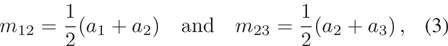respectively. The sequence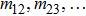of intermediate radii forms a geometric progression with the same ratio as that of the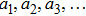sequence. Eliminating a 1 and a 3 between Equations (1) and (3), the Titius-Bode rule is transformed to the equivalent form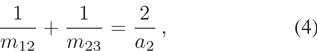which implies that a 2 is the harmonic mean of m 12 and m 23. As we describe in the Appendix, this is an important geometric property that is valid only in a central −1/r gravitational potential and its physical meaning can be easily deduced: Equation (4) can be rewritten in a form that can be interpreted in terms of central potential differences, viz.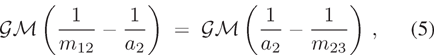where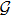is the gravitational constant and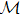is the mass of the central object that creates the gravitational field.

Consider now particles oscillating about the intermediate orbit O 2 (this also includes the protoplanetary core early in its formation and before it settles down to O 2). It is evident that the work done by a particle at m 12 to reach a 2 is the same as the work done by the field on a particle at m 23 that reaches a 2. In other words, the gravitational field allows orbit O 2 in Figure 1 to utilize the entire area between the hypothetical orbits through M 12 and M 23 and to accumulate matter while sharing with orbits O 1 and O 3 halfway the areas between each pair of adjacent orbits. This arrangement of orbits in a geometric progression ensures that adjacent orbits do not interfere with one another, a result that was first found by Laskar () who started with intersecting planitesimal orbits and derived the Titius-Bode rule for a surface density profile of the solar nebula when the interactions ceased. Our derivation above starts with the Titius-Bode rule and it is effectively the converse of Laskar’s derivation.

This “harmonic-mean” sharing by protoplanets of the in-between areas has also been used empirically in the seminal work of Weidenschilling () who distributed planetary material in annuli around the current orbits of planets in order to derive a surface density profile for the solar nebula. Our calculation justifies this empirical notion on energetic grounds: Equation (5) describes the energy balance of a harmonic oscillator in spherical (radial) coordinates with different amplitudes on either side of orbit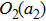and it is in contrast to the simple harmonic oscillator in which the deviations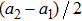and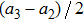from the equilibrium position a 2 are equal because of the linear nature of the restoring force (Hooke ).

## 3.1 Summary

We have described a physical interpretation of the Titius-Bode rule by considering, not the present positions of the planets in the solar system, but the “regions of occupancy” utilized by neighboring protoplanets during their efforts to collect and accumulate material as they orbit in the solar nebula: according to Equation (5), the work done by a particle to move out from an interior orbit through M 12 (Fig. 1) to the next outer planetary orbit O 2 is the same as the work done on a particle that falls into the gravitational field from M 23 to O 2.

The importance of adjacent protoplanets sharing halfway their in-between regions is twofold. First, the protoplanets do not cross into the orbits of their neighbors as they oscillate about their equilibrium orbits and continue to accumulate material (Laskar ; Jiang et al. ; Laskar & Petit ). This behavior ensures that some object or objects will be found in every single radial location, even in the predicted location between Mars and Jupiter (where the asteroid belt resides). Second, after the remaining disk gas disperses or gets accreted by the Sun and the planets emerge in their final settled orbits, the long-term dynamical stability of the solar system is strengthened because these orbits are as far away from one another as possible, and neighboring planets may interact only weakly by tidal forces that exert only minor perturbations on the positions of their neighbors (Hayes & Tremaine ). Such weak interactions are contingent upon the absence of resonant orbits, which is an observed fact for the planets in our solar system.

## 3.2 Solar Nebula

In Christodoulou & Kazanas (), we derived exact solutions of the Lane-Emden equations with rotation for the solar nebula (Lane ; Emden ) assuming it is an isothermal gas. The isothermal solutions of the Lane-Emden equations are very much relevant to the problem at hand: they show that protoplanetary cores are trapped inside local gravitational potential wells in which they can collect matter and grow in time. The distances of these localized potential wells from the protosun exhibit a geometric progression as a result of the differential rotation of the solar nebula (that tapers off at the inner region and at the farthest outer regions of the nebula, where the planetary orbits appear to follow arithmetic progressions).

The present result strengthens the argument that planets grow locally inside deep gravitational potential wells that extend halfway between adjacent planetary orbits: on energetic grounds, solid protoplanetary cores share the disk space in the solar nebula between adjacent orbits and they collect material by various processes that make matter settle down to the potential minima, whereas the gas can flow inward and continue its accretion on to the central protosun. Furthermore, this model argues against excessively large migrations of protoplanets in the solar nebula (Gomes et al. , ; Levison et al. , and references therein). Protoplanetary cores can move radially only within the bounds of their local gravitational potential wells (radii m 12 and m 23 in Eq. (3) for orbit O 2 in Fig. 1).

## 3.3 Extrasolar Multiplanet Systems

It is not surprising that at least some extrasolar systems exhibit similar characteristic distributions of exoplanetary orbits. Their protoplanetary disks may have had similar energetic and stability properties as our solar nebula, a similarity that apparently is neither universal nor wide-spread (Huang & Bakos ; Bovaird et al. ). As for the location of the habitable zone and its planets in extrasolar systems (Kane et al. ), we believe that the outcome depends crucially on the differential rotation and surface density profiles of each particular protoplanetary disk (Laskar ; Christodoulou & Kazanas ; Jiang et al. ) irrespective of whether the Titius-Bode rule is applicable or not.

## 3.4 Connection to the Closed Orbits of Bertrand’s Theorem

Equation (5) shows that perturbed particle orbits around a circular equilibrium orbit such asin Figure 1 have different amplitudes, say A 1 and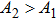, on either side of the equilibrium radius a 2. This is required so that the potential differences between a 2 and the maximum radial displacements be equal in magnitude, an assertion of the Work-Energy Theorem between the equilibrium radius a 2 and the radii of the turning points of the oscillation where the radial velocity goes to zero. The result is a restriction placed on the two amplitudes that must be related by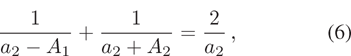that is, radius a 2 is the harmonic mean of the radii of the turning points. This property is valid only for bound closed orbits in a −1/r gravitational potential and it is derived in the Appendix, where we also analyze closed orbits in an r 2 gravitational potential (Bertrand ). It turns out that the latter orbits exhibit another precise symmetry altogether: radius a 2 is the geometric mean of the radii of the turning points.

Acknowledgements We thank the reviewers of this article for their comments that led to a clearer presentation of our ideas. DMC is obliged to Joel Tohline for advice and guidance over many years.

Appendix A: The Geometry of Bound Closed Orbits in Spherical Potentials
A1. Newton-Kepler −1/r Potential

Consider an equilibrium orbit r = a in a −1/r potential and assume that the maximum radial deviation is ±A on either side of r = a. At the turning points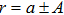, the radial velocity is zero (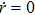) and the total energy per unit mass can then be written as (Goldstein )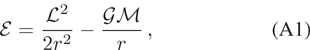where the specific angular momentum satisfies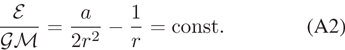Applied to the turning points, this equation yields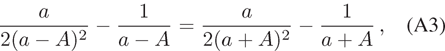a strict requirement for energy conservation. This requirement is satisfied only for A = 0 which implies that the amplitude of the oscillation cannot be the same on either side of r = a.

We consider next two different amplitudes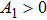andon either side of the equilibrium orbit r = a. After some elementary algebra, energy conservation (Eq. (A2)) at the turning points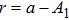and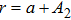yields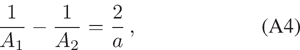or equivalently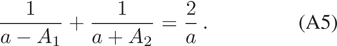This last equation shows that, in a −1/r potential, the equilibrium radius a is the harmonic mean of the radii of the turning points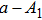and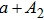(as was also found in Equation (4) for orbit O 2, and points M 12 and M 23 in Fig. 1).

A2. Isotropic Hooke r 2 Potential

The isotropic harmonic-oscillator potential, written as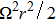(Ω=const.), cannot support arbitrarily large oscillations of equal amplitude on either side of the equilibrium orbit r = a either. The same analysis leads to an energy equation analogous to Equation (A2), but here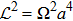, thus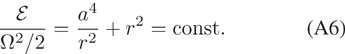When energy conservation is applied between the turning points, we obtain three solutions, A = 0 and two extraneous solutions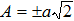. The solution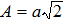is of course rejected because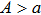.

We consider next two different amplitudesandon either side of the equilibrium orbit r = a. After some elementary algebra, energy conservation (Eq. (A6)) at the turning points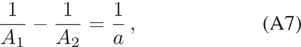or equivalently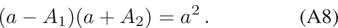This last equation shows that, in a harmonic r 2 potential, the equilibrium radius a is the geometric mean of the radii of the turning pointsand.

 Bertrand J. 1873 C. R. Acad. Sci. Paris 77 849 Bovaird T. Lineweaver C. H. 2013 MNRAS 435 1126 Bovaird T. Lineweaver C. H. Jacobsen S. K. 2015 MNRAS 448 3608 Christodoulou, D.M., & Kazanas, D., 2007. arXiv:0706.3205. Danby J. M. A. 1988 Fundamentals of Celestial Mechanics 2 Richmond, Va., U.S.A Willmann-Bell Dubrulle B. Graner F. 1994 A&A 282 269 Emden R. 1907 Gaskugeln Leipzig B. G. Teubner https://en.wikipedia.org/wiki/Robert_Emden) Goldstein H. 1950 Classical Mechanics Mass Addison-Wesley (Addison-Wesley World Student Series, Reading) Gomes R. S. Morbidelli A. Levison H. F. 2004 Icarus 170 492 Gomes R. S. Gallardo T. Fernández J. A. Brunini A. 2005 Celest. Mech. Dyn. Astron. 91 109 Graner F. Dubrulle B. 1994 A&A 282 262 Hayes W. Tremaine S. 1998 Icarus 135 549 Hooke R. 1678 De Potentia Restitutiva, or of Spring. Explaining the Power of Springing Bodies London J. Martyn Huang C. X. Bakos G Á. 2014 MNRAS 442 674 Jaki S. 1972 Am. J. Phys. 40 93 Jiang I-G. Yeh L-C. Hung W-L. 2015 MNRAS 449 L65 Kane S. R. Hill M. L. Kasting J. F. 2016 ApJ 830 1 Lane L J H 1870 Amer. J. Sci. Arts, Second Series 50 57 Laskar J. 2000 Phys. Rev. Lett. 84 3240 Laskar J. Petit A. C. 2017 A&A 605 A72 Lecar M. 1973 Nature 242 318 Levison H. F. Morbidelli A. Gomes R. Backman D. 2007 Protostars and Planets V 669 Li X. Q. Zhang H. Li Q. B. 1995 A&A 304 617 Lynch P. 2003 MNRAS 341 1174 Murray C. D. Dermott S. F. 1999 Solar system dynamics Cambridge Cambridge Univ. Press Neuhaeuser R. Feitzinger J. V. 1986 A&A 170 174 Nieto M. M. 1972 The Titius-Bode Law of Planetary Distances: Its History and Theory Oxford Pergamon Press Nottale L. Schumacher G. Gay J. 1997 A&A 322 1018 Poveda A. Lara P. 2008 Revista Mexicana de Astronomia y Astrofisica Conference Series 34 49 Poveda A. Lara P. 2008 RMxAA 44 243 Weidenschilling S. J. 1977 Ap&SS 51 153
Cite this article: Christodoulou Dimitris M., Kazanas Demosthenes. A physical interpretation of the Titius-Bode rule and its connection to the closed orbits of Bertrandʼs theorem. Res. Astron. Astrophys. 2017; 12:129.

### Refbacks

• There are currently no refbacks.